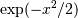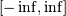# numpy.polynomial.hermite_e.hermeweight¶

numpy.polynomial.hermite_e.hermeweight(x)[source]

Weight function of the Hermite_e polynomials.

The weight function isand the interval of integration is. the HermiteE polynomials are orthogonal, but not normalized, with respect to this weight function.

Parameters: x : array_like Values at which the weight function will be computed. w : ndarray The weight function at x.

Notes

#### Previous topic

numpy.polynomial.hermite_e.hermegauss

#### Next topic

numpy.polynomial.hermite_e.hermecompanion